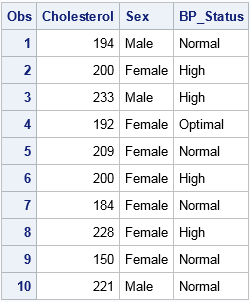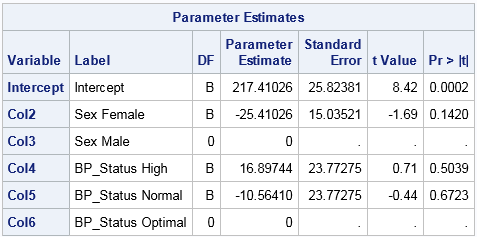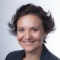A dummy variable (also known as indicator variable) is a numeric variable that indicates the presence or absence of some level of a categorical variable. The word "dummy" does not imply that these variables are not smart. Rather, dummy variables serve as a substitute or a proxy for a categorical variable, just as a "crash-test dummy" is a substitute for a crash victim, or a "sewing dummy" is a dressmaker's proxy for the human body.

In regression and other statistical analyses, a categorical variable can be replaced by dummy variables. For example, a categorical variable with levels "Low," "Moderate," and "High" can be represented by using three binary dummy variables. The first dummy variable has the value 1 for observations that have the level "Low," and 0 for the other observations. The second dummy variable has the value 1 for observations that have the level "Moderate," and zero for the others. The third dummy variable encodes the "High" level.

There are many ways to construct dummy variables in SAS. Some programmers use the DATA step, but there is an easier way. This article discusses the GLMMOD procedure, which produces basic binary dummy variables. A follow-up article discusses other SAS procedures that create a design matrix for representating categorical variables.

### Why generate dummy variables in SAS?

Many programmers never have to generate dummy variables in SAS because most SAS procedures that model categorical variables contain a CLASS statement. If a procedure contains a CLASS statement, then the procedure will automatically create and use dummy variables as part of the analysis.

However, it can be useful to create a SAS data set that explicitly contains a design matrix, which is a numerical matrix that use dummy variables to represent categorical variables. A design matrix also includes columns for continuous variables, the intercept term, and interaction effects. A few reasons to generate a design matrix are:

• Students might need to create a design matrix so that they can fully understand the connections between regression models and matrix computations.
• If a SAS procedure does not support a CLASS statement, you can use often use dummy variables in place of a classification variable. An example is PROC REG, which does not support the CLASS statement, although for most regression analyses you can use PROC GLM or PROC GLMSELECT. Another example is the MCMC procedure, whose documentation includes an example that creates a design matrix for a Bayesian regression model.
• In simulation studies of regression models, it is easy to generate responses by using matrix computations with a numerical design matrix. It is harder to use classification variables directly.

### PROC GLMMOD: Design matrices that use the GLM parameterization

The following DATA step create a data set with 10 observations. It has one continuous variable (Cholesterol) and two categorical variables. One categorical variable (Sex) has two levels and the other (BP_Status) has three levels.

```data Patients; keep Cholesterol Sex BP_Status; set sashelp.heart; if 18 <= _N_ <= 27; run;   proc print; var Cholesterol Sex BP_Status; run;```The GLMMOD procedure can create dummy variables for each categorical variable. If a categorical variable contains k levels, the GLMMOD procedure creates k binary dummy variables. The GLMMOD procedure uses a syntax that is identical to the MODEL statement in PROC GLM, so it is very easy to use to create interaction effects.

The following call to PROC GLMMOD creates an output data set that contains the dummy variables. The output data set is named by using the OUTDESIGN= option. The OUTPARAM= option creates a second data set that associates each dummy variable to a level of a categorical variable:

```proc glmmod data=Patients outdesign=GLMDesign outparm=GLMParm; class sex BP_Status; model Cholesterol = Sex BP_Status; run;   proc print data=GLMDesign; run; proc print data=GLMParm; run;```The OUTDESIGN= data set contains the design matrix, which includes variables named COL1, COL2, COL3, and so forth. The OUTPARM= data set associates levels of the original variables to the dummy variables. For these data, the GLMMOD procedure creates six binary columns. The first is the intercept column. The next two encode the Sex variable. The last three encode the BP_Status variable. If you specify interactions between the original variables, additional dummy variables are created. Notice that the order of the columns is the sort order of the values of their levels. For example, the "Female" column appears before the "Male" column.

When you use this design matrix in a regression analysis, the parameter estimates of main effects estimate the difference in the effects of each level compared to the last level (in alphabetical order). The following statements show that using the dummy variables in PROC REG give the same parameter estimates as are obtained by using the original classification variables in PROC GLM:

```ods graphics off; /* regression analysis by using dummy variables */ proc reg data=GLMDesign; DummyVars: model Cholesterol = COL2-COL6; /* dummy variables except intercept */ ods select ParameterEstimates; quit;   /* same analysis by using the CLASS statement */ proc glm data=Patients; class sex BP_Status; /* generates dummy variables internally */ model Cholesterol = Sex BP_Status / solution; ods select ParameterEstimates; quit;```The parameter estimates from PROC REG is shown. The parameter estimates from PROC GLM are identical. Notice that the parameter estimates for the last level are set to zero and the standard errors are assigned missing values. This occurs because the dummy variable for each categorical variable is redundant. For example, the second dummy variable for the Sex variable ("Males") is a linear combination of the intercept column and the dummy variable for "Females"). Similarly, the last dummy variable for the BP_Status variable ("Optimal") is a linear combination of the intercept column and the "High" and "Normal" dummy variables. By setting the parameter estimate to zero, the last column for each set of dummy variables does not contribute to the model.

For this reason, the GLM encoding is called a singular parameterization. In my next blog post I will present ways to parameterize levels of the categorical variables that lead to nonsingular design matrices.

Share

### About AuthorDistinguished Researcher in Computational Statistics

Rick Wicklin, PhD, is a distinguished researcher in computational statistics at SAS and is a principal developer of PROC IML and SAS/IML Studio. His areas of expertise include computational statistics, simulation, statistical graphics, and modern methods in statistical data analysis. Rick is author of the books Statistical Programming with SAS/IML Software and Simulating Data with SAS.

### 20 Comments

1.Neat! My last new thing I learned today. :-)

Reminds me of the blog post series Chris Daman wrote a few years ago on GLM and reference cell coding. Might provide some background information.

•Yes, Chris and many other SAS programmers use the DATA step to create dummy variables. Using the DATA step is cumbersome and prone to errors, so I much prefer using the SAS procedures that can create dummy variables.

•Rick,
"Using the DATA step is cumbersome and prone to errors, "
I don't agree with that. I think data step is beautiful ,fast and more powerful than your method.
But good to know the statistical explanation of WHY " the GLM encoding is called a singular parameterization " .

•Yes, the DATA step is fast and beautiful, and for the simple example in this post I'm sure that you could write the analogous DATA step quickly and correctly. However, for categorical variable with many levels and for models that have complex interactions, procedures are easier to use and have been thoroughly tested for correctness. For example, here is a regression model that involves all main effects and two-way interactions for four categorical variables. The design matrix has 345 columns, but you can generate it with only four statements. The corresponding DATA step/macro code would be much longer and more difficult to write. For an example, see Tian (2004).

```proc glmmod data=sashelp.cars outdesign=BigDesign; class cylinders make origin type; model mpg_city = cylinders | make | origin | type @ 2; /* main effects and 2-way interactions */ run;```
2.Very nice tip. At first I thought, "well, I won't use this because I need meaningful var names, not COL1 COL2 etc. And I don't want to have the map the OUTDESIGN= data back to the OUTPARM= data." But then I realized the variable labels in OUTDESIGN= data have just what I would want to make meaningful var names. Good deal.

•Thanks for pointing that out. Quentin is referring to the first two columns of the parameter estimates table from PROC REG. The first column lists the variable names as COL2-COL6, but the second column lists the informative labels.

3.Just when I'm studying Data Mining! Rick, once again you have proved that you are an indispensable component of the SAS ecosystem.

Thanks!
Tom

•I aim to please :-) Glad you find it useful.

4.How can I simulate categorical variables that would not be redundant? That is the dummy variables would not be a linear combination of the intercept column.

•I think you are talking about nonsingular design matrices such as produced by using the REFERENCE or EFFECT encodings. See the link in the last sentence of this article.

5.This is really helpful when wanting to use Proc Reg and do VIF or TOL or other diagnostics that seem to be more difficult in Proc GLM. However, is there an easy way to return the variable names back to what they were before? Or to transfer the variable labels into what they are labeled as? I found this but can't seem to get it to work without the error: "Literal contains unmatched quote" http://support.sas.com/kb/24/720.html

•I don't know what you mean by "return the variable names back to what they were before," but maybe your question is about whether you can generate a design matrix that has informative names instead of COL1, COL2, etc. Yes, you can generate names that are informative.

6.thank you so much! this was extremely helpful to create my dummy variables and saved me a great amount of time

7.Hi! Thank you very much for this helpful explanation! Is there a way to change the reference level in the GLMMOD procedure?

•Not in PROC GLMMOD, but click on the link in the last sentence to see another article. In that article, I describe other SAS procedures for which you can set the reference level.